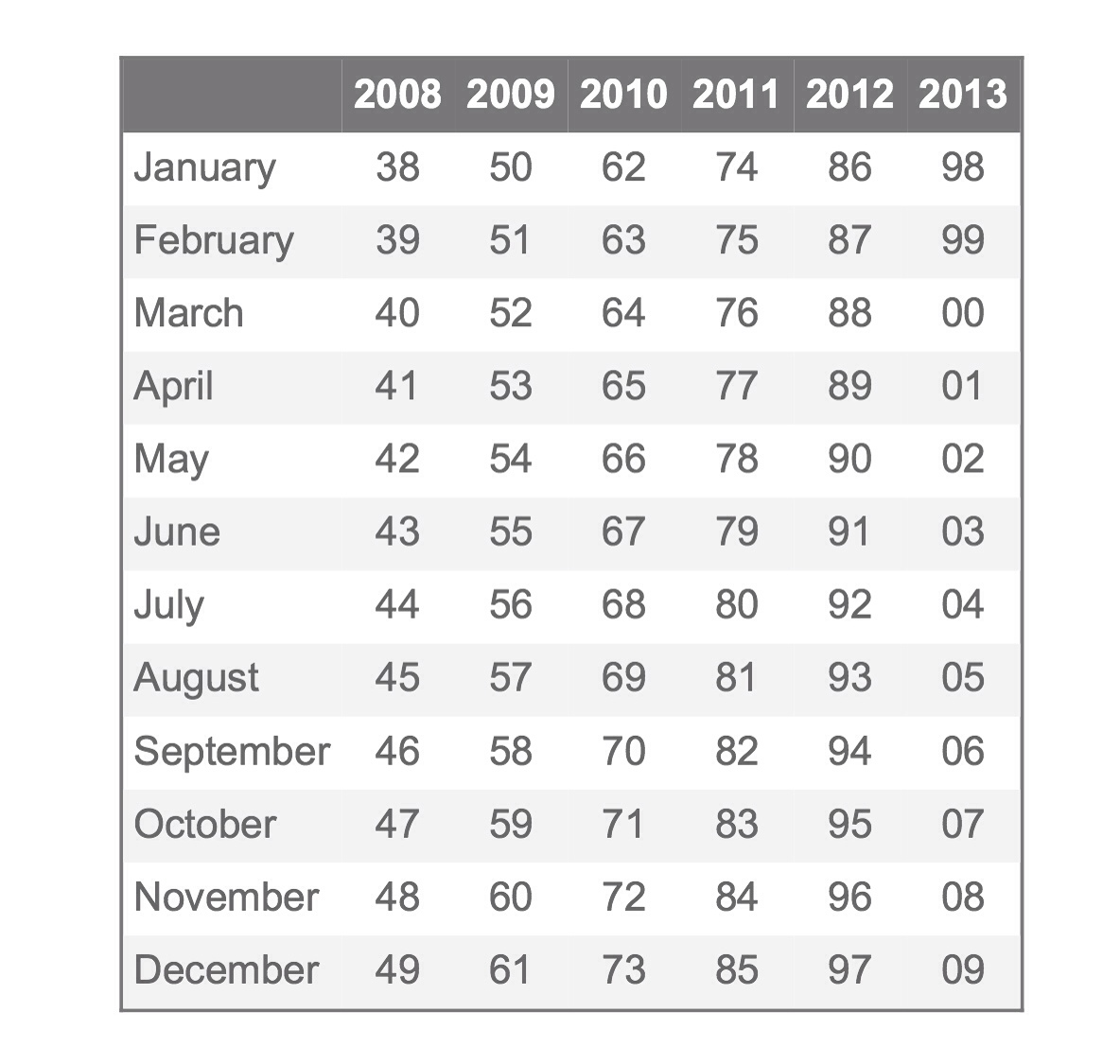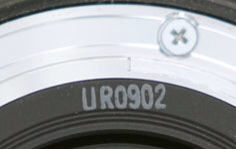# Want To Know How Old Your Canon Lens Is?Image credit: The Digital Picture

Instructive post by The Digital Picture. How to know the age of your Canon glass? First, let's quote The Digital Picture's post:

Canon has been transitioning to a 10-digit lens serial number (starting in 2008 with the Canon EF-S 18-200mm f/3.5-5.6 IS Lens) and ending the inclusion of a separate manufacturing date code. While date codes and the shorter serial number are still found on some lenses manufactured as late as 2012, this inclusion will likely end completely. We loved the date code because it made aging a lens easy. However, now we can age a lens based on the serial number alone.

So, want to know the age of a lens using the 10 digits serial numbers? No problem. First, split the serial number in three parts: DD C SSSSSSS. The “DD” part mens the year of manufacturing (see image above). Be aware that the code assigned to the year 2013 is a prediction, the code may also become “DDD”. The “C” indicates the batch, the “SSSSSSS” is the serial number assigned to the lens.

And what about lenses made before the 10 digits system? Check the date code beside the rear lens element, see image below.Image credit: The Digital Picture

The first letter (“U” in the image) indicates the factory where the lens was made. “U” stands for Utsunomiya, “F” for Fukushima and “O” for Oita, all in Japan. The second letter (“R” in the image above) is the code of the year of manufacturing. See below:

• A = 2012, 1986, 1960
• B = 1987, 1961
• C = 1988, 1962
• D = 1989, 1963
• E = 1990, 1964
• F = 1991, 1965
• G = 1992, 1966
• H = 1993, 1967
• I = 1994, 1968
• J = 1995, 1969
• K = 1996, 1970
• L = 1997, 1971
• M = 1998, 1972
• N = 1999, 1973
• O = 2000, 1974
• P = 2001, 1975
• Q = 2002, 1976
• R = 2003, 1977
• S = 2004, 1978
• T = 2005, 1979
• U = 2006, 1980
• V = 2007, 1981
• W = 2008, 1982
• X = 2009, 1983
• Y = 2010, 1984
• Z = 2011, 1985

Canon incremented this letter each year, starting with A in 1986 and before using A in 1960 (without the initial factory code).

Finally, the first two numbers (“09” in the example) mean the month of manufacturing, the final two numbers (“02” in the example) are an internal code used by Canon. Sometimes the month code comes without the leading zero.

[thanks to The Digital Picture]